# Perimeter - 8th grade (13y) - math problems

#### Number of problems found: 219

• Perimeter of the circleCalculate the perimeter of the circle in dm, whose radius equals the side of the square containing 0.49 dm2?
• Square - increased perimeterHow many times is increased perimeter of the square, where its sides increases by 150%? If the perimeter of square will increase twice, how much% increases the content area of the square?
• Perimeter of a rectangleIf the perimeter of a rectangle is 114 meters and the length is twice the width plus 6 meters, what are the length and width?
• Parallelogram perimeter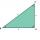The ABC triangle with sides a = 5cm, b = 3cm, c = 40mm has the center of the sides of the K,L,M. How many cm have the KBLM parallelogram perimeter?
• Cross five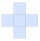The figure on the picture is composed of the same squares and has a content of 45cm². What's its perimeter?
• Perimeter and legsDetermine the perimeter of a right triangle if the length of one leg is 75% length of the second leg and its content area is 24 cm2.
• HeightCalculate height of the equilateral triangle if its perimeter is 8?
• Find theFind the radius of the circular base of the vessel, whose perimeter is 2.51 m. Write the result to one decimal place in meters. Via the π sign - Ludolph's number
• Area to perimeterCalculate circle circumference if its area is 254.34cm2Determine the radius of the circle, if its perimeter and area is the same number.
• RT 11Calculate the area of right tirangle if its perimeter is p = 45 m and one cathethus is 20 m long.
• Trapezoid ABCD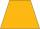Calculate the perimeter of trapezoid ABCD if we know the side c=15, b=19 which is also a height and side d=20.
• Perimeter of circleCalculate the circumference of described circle to the triangle with sides 9,12,15 cm.
• Equilateral triangle v2Equilateral triangle has a perimeter 36 dm. What is its area?
• In aIn a triangle, the aspect ratio a: c is 3: 2 and a: b is 5: 4. The perimeter of the triangle is 74cm. Calculate the lengths of the individual sides.
• Rectangle - sides 4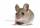Perimeter of the rectangle is 72 cm. Calculate the length of the sides that are in the ratio 3:5.
• ParallelogramThe parallelogram has sides lengths in the ratio 3: 4 and perimeter 2.8 meters. Determine the lengths of the sides.
• A rectangle's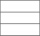A rectangle's length is 3 times more than twice its width. If the perimeter of the rectangle is 60 feet. What is the length of the rectangle
• The sidesThe sides of a rectangle are in a ratio of 2:3, and its perimeter is 1 1/4 inches. What are the lengths of its side? Draw it.
• Triangle SAAThe triangle has one side long 71 m and its two internal angles is 60°. Calculate the perimeter and area of the triangle.

Do you have an interesting mathematical word problem that you can't solve it? Submit a math problem, and we can try to solve it.

We will send a solution to your e-mail address. Solved examples are also published here. Please enter the e-mail correctly and check whether you don't have a full mailbox.

Please do not submit problems from current active competitions such as Mathematical Olympiad, correspondence seminars etc...